Project in progress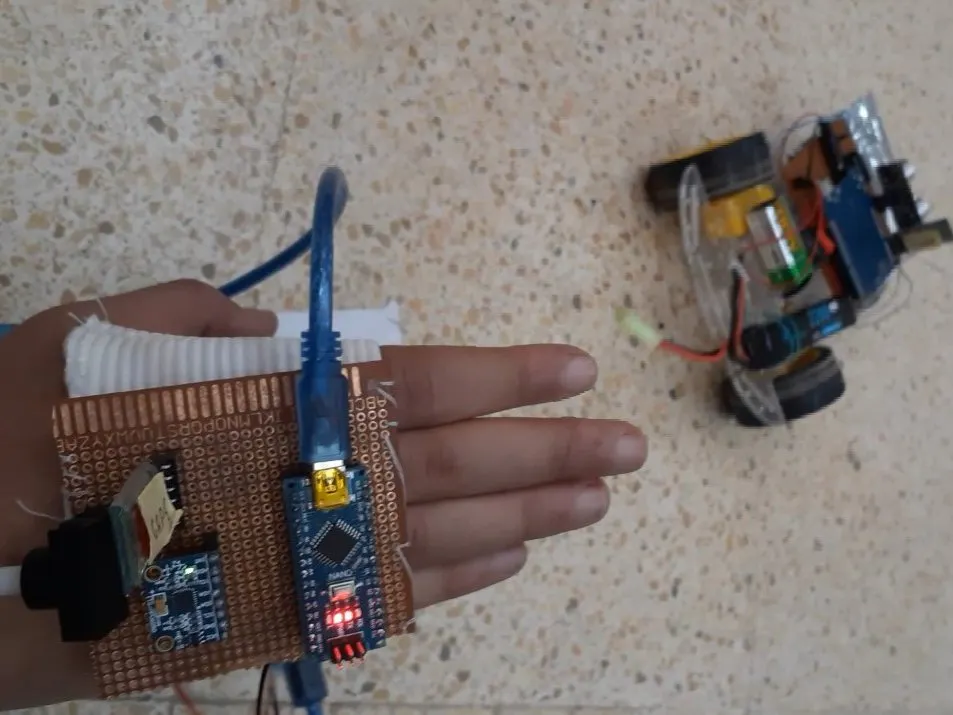# Hand Gesture Controlled robot

Make this robot moves by just moving your hand!

• 67 views
• 1 respect

## Components and suppliesArduino UNO
×1Arduino Nano R3
×1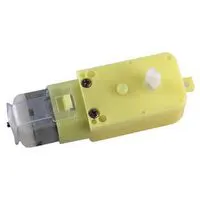DC Motor, 12 V
×1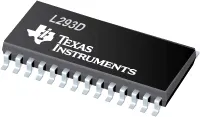Texas Instruments Dual H-Bridge motor drivers L293D
×1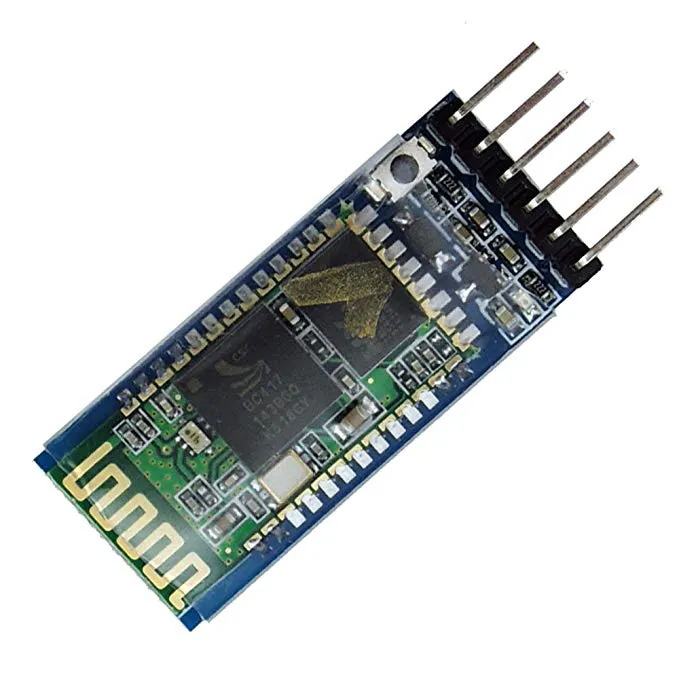HC-05 Bluetooth Module
×1Ultrasonic Sensor - HC-SR04 (Generic)
×1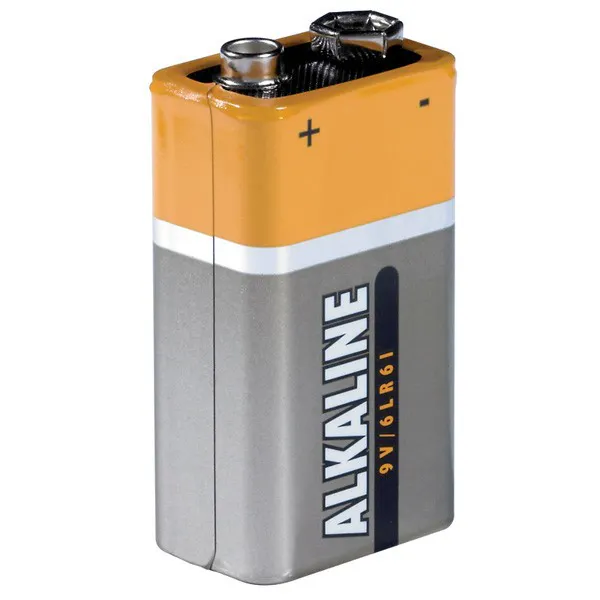9V battery (generic)
×1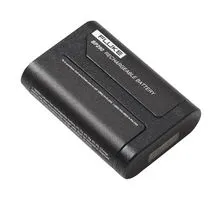Rechargeable Battery, Lithium Ion
×1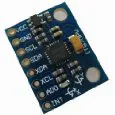Inertial Measurement Unit (IMU) (6 deg of freedom)
×1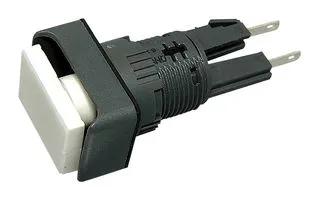Switch Actuator, APEM A01 series Illuminated Push-Button Switches
×1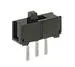Slide Switch
×1
 PCB's
×1
 Robot wheels
×1
 Robot chassis
×1

## Apps and online services

### INTRO:

The ability to control things by a hand seen in movies was like magic, I was so excited to know how it works and try it in real life. So I come out with a very nice project which is a car that can be controlled by moving your hand in the direction you want and the car will follow! it's a cool project and very simple!

This project is essentially made of two-part, the transmission station placed on the hand and the reception one which is the car.

### The transmission unit:

Contains Arduino nano (not heavy to handle)

mpu6050 sensor

Bluetooth HC-05 module(master)

push-button, battery 9v.

All components are soldering in the PCB board.

The MPU6050 module is made of two built-in sensors which are the gyroscope and the accelerometer, each of these sensors gives data and gets processed by the Arduino nano in order to get the appropriate angle, then according to the output data we'll send special characters that specify the direction the car will choose via Bluetooth module to the Arduino UNO in the car. The code for the transmission unit has been provided in the latter part of this project.

The logic I've used :

If the value along the x-axis is under -17, then it should send 'F'.

• If the value along the x-axis is above 20, then it should send 'B'.
• If the value along the y-axis is above 30, then it should send 'R'.
• If the value is under-30, then it should send 'L'.
• If none of the above conditions are satisfied, then it should send 'S'.

You will notice that I obtain yaw data but I didn't use it to make the hand easy to use (OPTIONAL)

### The reception unit:

basically made of an Arduino UNO

Bluetooth module(slave):receiving data.

H-bridge l293d: control direction and speed of motors.

9v battery to power the Arduino UNO and other components.

12v to power the motors.

Ultrasonic sensor: an additional sensor to avoid crashes.

All components are soldering in the PCB board.

After receiving the data(special characters), and according to the code written for the Arduino UNO the car will move.

The logic I've used:

If the reception unit receives the character 'F',then the robot should move forward.

• If 'B', then the robot should move backward.
• If 'R', then the robot should move right.
• If 'L', then the robot should move left.
• If 'S', then the robot should stop.

### Some Troubleshooting:

Sometimes your robot will not move in the correct direction. in this case try to find out which motor behaves in this way. Then you can adjust it from the code or just interchanged the wires of the motors.

### It works!

if you face any problems with this project just let me know in the comment section.

## Code

##### Transmitter codeArduino
Upload it to the transmission unit.
```/*
Arduino and MPU6050 Accelerometer and Gyroscope Sensor Tutorial
by Dejan, https://howtomechatronics.com/tutorials/arduino/arduino-and-mpu6050-accelerometer-and-gyroscope-tutorial/
*/

#include <Wire.h>
#include <SoftwareSerial.h>
SoftwareSerial BTSerial(10, 11); // CONNECT BT RX PIN TO ARDUINO 11 PIN | CONNECT BT TX PIN TO ARDUINO 10 PIN

float AccX, AccY, AccZ;
float GyroX, GyroY, GyroZ;

float accAngleX, accAngleY, gyroAngleX, gyroAngleY, gyroAngleZ;
float roll, pitch, yaw;

float AccErrorX, AccErrorY, GyroErrorX, GyroErrorY, GyroErrorZ;

float elapsedTime, currentTime, previousTime;

int c = 0;

void setup()
{

Serial.begin(9600);
BTSerial.begin(9600);  // HC-05 default speed in AT command more

Wire.begin();                      // Initialize comunication
Wire.beginTransmission(MPU_ADDRESS);       // Start communication with MPU6050 // MPU=0x68
Wire.write(0x6B);                  // Talk to the register 6B
Wire.write(0x00);                  // Make reset - place a 0 into the 6B register
Wire.endTransmission(true);        //end the transmission

// Configure Accelerometer Sensitivity - Full Scale Range (default +/- 2g)
/*  Wire.beginTransmission(MPU);
Wire.write(0x1C);                  //Talk to the ACCEL_CONFIG register (1C hex)
Wire.write(0x10);                  //Set the register bits as 00010000 (+/- 8g full scale range)
Wire.endTransmission(true);

// Configure Gyro Sensitivity - Full Scale Range (default +/- 250deg/s)
Wire.beginTransmission(MPU);
Wire.write(0x1B);                   // Talk to the GYRO_CONFIG register (1B hex)
Wire.write(0x10);                   // Set the register bits as 00010000 (1000deg/s full scale)
Wire.endTransmission(true);
delay(20);

//Call this function if you need to get the IMU error values for your module*/
//while(true) calculate_IMU_error();

// delay(20);

}

void loop()
{

// calculate time elapsed since last time we were here
previousTime = currentTime;        // Previous time is stored before the actual time read
currentTime = millis();            // Current time actual time read
elapsedTime = (currentTime - previousTime) / 1000; // Divide by 1000 to get seconds

// Complementary filter - combine acceleromter and gyro angle values
roll  = 0.92 * (roll  + (GyroX * elapsedTime)) + 0.08 * accAngleX;
pitch = 0.92 * (pitch + (GyroY * elapsedTime)) + 0.08 * accAngleY;
//yaw   = gyroAngleZ;

// Currently the raw values are in degrees per seconds, deg/s, so we need to multiply by sendonds (s) to get the angle in degrees
gyroAngleX += GyroX * elapsedTime; // deg/s * s = deg
gyroAngleY += GyroY * elapsedTime;
gyroAngleZ += GyroZ * elapsedTime;

/*by me */
// Print the values on the serial monitor
Serial.print("roll:");
Serial.print(roll);
Serial.print("    ");

Serial.print("pitch:");
Serial.println(pitch);

/* Serial.print("    ");
Serial.print("yaw:");
Serial.println(yaw);*/

/*Serial.print(" ");
// Print the values on the serial monitor
Serial.print(pitch);
Serial.print(" ");
Serial.print(accAngleY);
Serial.print(" ");
Serial.println(gyroAngleY);
*/
if(pitch<-17){
BTSerial.write('F');
}else if(pitch>20){
BTSerial.write('B');
}else if(roll>30){
BTSerial.write('R');
}else if(roll<-30){
BTSerial.write('L');
}else if(yaw>30){
BTSerial.write('r');
}else if(yaw<-30){
BTSerial.write('l');
}else
BTSerial.write('S');
}

{
// === Read acceleromter data === //
Wire.endTransmission(false);
Wire.requestFrom(MPU_ADDRESS, 6, true); // Read 6 registers total, each axis value is stored in 2 registers

//For a range of +-2g, we need to divide the raw values by 16384, according to the datasheet
AccX = (Wire.read() << 8 | Wire.read()) / 16384.0; // X-axis value
AccY = (Wire.read() << 8 | Wire.read()) / 16384.0; // Y-axis value
AccZ = (Wire.read() << 8 | Wire.read()) / 16384.0; // Z-axis value

// Calculating Roll and Pitch from the accelerometer data
accAngleX = (atan(AccY / sqrt(pow(AccX, 2) + pow(AccZ, 2))) * 180 / PI)      - (-0.40);  // AccErrorX ~(0.58) See the calculate_IMU_error()custom function for more details
accAngleY = (atan(-1 * AccX / sqrt(pow(AccY, 2) + pow(AccZ, 2))) * 180 / PI) - (-3.75); // AccErrorY ~(-1.58)

}

{
// === Read gyroscope data === //
Wire.write(0x43); // Gyro data first register address 0x43
Wire.endTransmission(false);
Wire.requestFrom(MPU_ADDRESS, 6, true); // Read 4 registers total, each axis value is stored in 2 registers

// For a 250deg/s range we have to divide first the raw value by 131.0, according to the datasheet

// Correct the outputs with the calculated error values
GyroX = GyroX - (-0.68); // GyroErrorX = ~ (-2.12)
GyroY = GyroY - (-2.48);  // GyroErrorY = ~ (4.12)
GyroZ = GyroZ - (-0.12);  // GyroErrorZ = ~ (1.20)

}

void calculate_IMU_error()
{

// We can call this funtion in the setup section to calculate the accelerometer and gyro data error. From here we will get the error values used in the above equations printed on the Serial Monitor.
// Note that we should place the IMU flat in order to get the proper values, so that we then can the correct values
// Read accelerometer values 200 times
while (c < 200)
{
Wire.write(0x3B);
Wire.endTransmission(false);
AccErrorX = AccErrorX + ((atan((AccY) / sqrt(pow((AccX), 2) + pow((AccZ), 2))) * 180 / PI));
AccErrorY = AccErrorY + ((atan(-1 * (AccX) / sqrt(pow((AccY), 2) + pow((AccZ), 2))) * 180 / PI));
c++;
}

//Divide the sum by 200 to get the error value
AccErrorX = AccErrorX / 200;
AccErrorY = AccErrorY / 200;

c = 0;

// Read gyro values 200 times
while (c < 200)
{
Wire.write(0x43);
Wire.endTransmission(false);

GyroErrorX = GyroErrorX + (GyroX / 131.0);
GyroErrorY = GyroErrorY + (GyroY / 131.0);
GyroErrorZ = GyroErrorZ + (GyroZ / 131.0);

c++;

}

//Divide the sum by 200 to get the error value
GyroErrorX = GyroErrorX / 200;
GyroErrorY = GyroErrorY / 200;
GyroErrorZ = GyroErrorZ / 200;

// Print the error values on the Serial Monitor

Serial.print("AccErrorX: ");
Serial.print(AccErrorX);
Serial.print(" | AccErrorY: ");
Serial.print(AccErrorY);
Serial.print(" | GyroErrorX: ");
Serial.print(GyroErrorX);
Serial.print(" | GyroErrorY: ");
Serial.print(GyroErrorY);
Serial.print(" | GyroErrorZ: ");
Serial.println(GyroErrorZ);

delay(1000);
}
```
Upload it code to the reception unit
```#include <SoftwareSerial.h>

SoftwareSerial BTSerial(10, 11); // CONNECT BT RX PIN TO ARDUINO 12 PIN | CONNECT BT TX PIN TO ARDUINO 11 PIN
#include<SPI.h>

int trigPin = 12;    // Trig
int echoPin = 13;    // Echo
long duration, cm, inches;
int direct;
int l1=3;
int r1=5;
int l2=6;
int r2=9;

int forword_corr;

int a = 0 ;
int s = 3;

int b = 0 ;
int c = 2;

void setup() {
Serial.begin(9600);
BTSerial.begin(9600);

pinMode(l1, OUTPUT);
pinMode(r1, OUTPUT);
pinMode(l2, OUTPUT);
pinMode(r2, OUTPUT);

digitalWrite(l1, LOW);
digitalWrite(r1, LOW);
digitalWrite(l2, LOW);
digitalWrite(r2, LOW);

pinMode(trigPin, OUTPUT);
pinMode(echoPin, INPUT);
}

void loop() {

digitalWrite(trigPin, LOW);
delayMicroseconds(5);
digitalWrite(trigPin, HIGH);
delayMicroseconds(10);
digitalWrite(trigPin, LOW);

pinMode(echoPin, INPUT);
duration = pulseIn(echoPin, HIGH);

cm = (duration/2) / 29.1;     // Divide by 29.1 or multiply by 0.0343
Serial.print(cm);
Serial.print("cm");
Serial.println();
if(BTSerial.available()>0){ // Witing for data incoming from the other XBee module

if(direct == 'B'){
Serial.println("Backward");
backward();
}else if (direct=='F'){
Serial.println("Forward");
forward();
}else if(direct == 'R'){
Serial.println("Right");
left();
}else if(direct == 'L'){
Serial.println("left");
right();

}else if(direct == 'r'){
Serial.println("rotright");
rotr();

}else if(direct == 'l'){
Serial.println("rotleft");
rotl();
}else if  (direct == 'S'){
Serial.println("Stop");
stopCar();
}

}
}

void forward()
{
if(cm<=15){
digitalWrite(l1, LOW);
digitalWrite(r2, LOW);

}
else{
digitalWrite(l1, HIGH);
digitalWrite(r1, LOW);
digitalWrite(l2, LOW);

digitalWrite(r2, LOW);
delay(2);
digitalWrite(r2, HIGH);
delay(2);
}
}
void backward()
{
digitalWrite(l1, LOW);
digitalWrite(l2, HIGH);
digitalWrite(r2, LOW);
if(b%c == 0){
digitalWrite(r1, HIGH);
}else{
digitalWrite(r1, LOW);
}

if(b > 1000 ) {
b=0;
}
}
void right()
{
digitalWrite(l1, HIGH);
digitalWrite(r1, LOW);
digitalWrite(l2, LOW);
analogWrite(r2, 100);

}
void left()
{
analogWrite(l1, 100);
digitalWrite(r1, LOW);
digitalWrite(l2, LOW);
digitalWrite(r2, HIGH);
}

void stopCar() {
digitalWrite(l1, LOW);

digitalWrite(r1, LOW);
digitalWrite(l2, LOW);
digitalWrite(r2, LOW);
}
void rotr() {

digitalWrite(l1, HIGH);
digitalWrite(r1, LOW);
digitalWrite(l2, HIGH);
digitalWrite(r2, LOW);
}
void rotl(){

digitalWrite(l1, LOW);
digitalWrite(r1, HIGH);
digitalWrite(l2, LOW);
digitalWrite(r2, HIGH);
}
```

## Schematics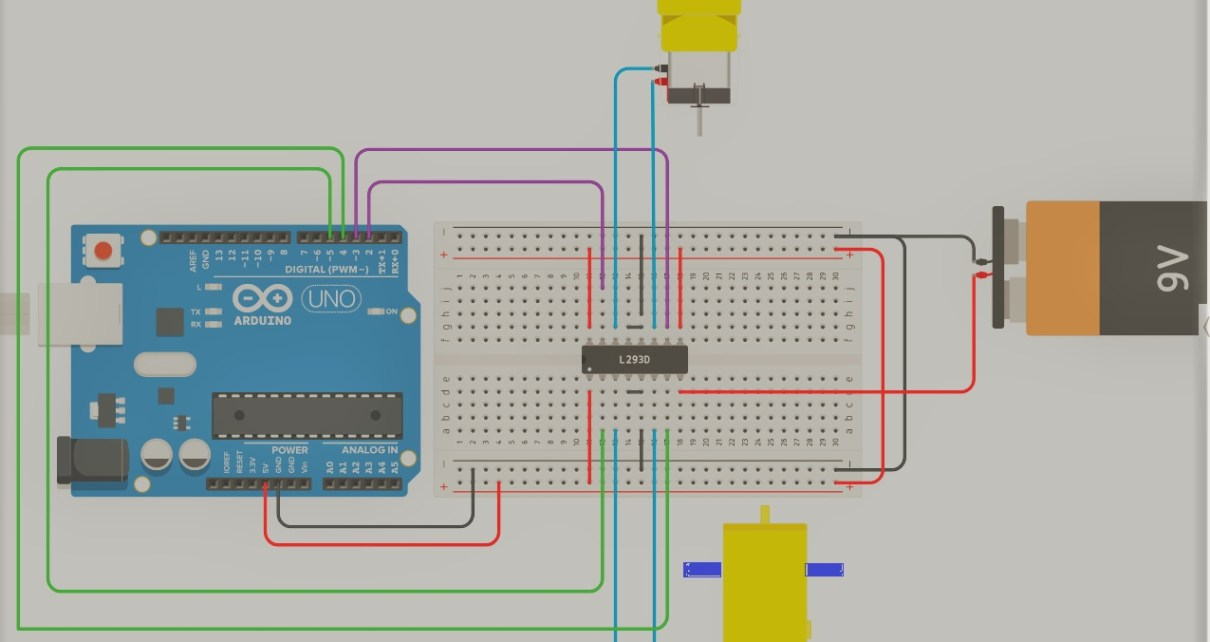August 26, 2021

#### Members who respect this project

See similar projects
you might like

#### Gesture controlled robot

Project tutorial by Harish K and Ashwin S

• 6,543 views
• 8 respects

#### How to Make a Gesture Control Robot at Home

Project tutorial by Shubham Shinganapure

• 32,619 views
• 94 respects

#### DIY Arduino Robot Arm – Controlled by Hand Gestures

Project tutorial by Eben Kouao

• 16,720 views
• 48 respects

#### Hand Gesture Controlled Robot

Project tutorial by Mayoogh Girish

• 109,503 views
• 118 respects

#### Simple Gesture Controlled Robot Using Arduino

Project tutorial by Jithin Sanal

• 17,476 views